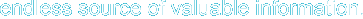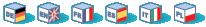# Chapter 15. Operators

Operator Precedence
Arithmetic Operators
Assignment Operators
Bitwise Operators
Comparison Operators
Error Control Operators
Execution Operators
Incrementing/Decrementing Operators
Logical Operators
String Operators
Array Operators
Type Operators

An operator is something that you feed with one or more values (or expressions, in programming jargon) which yields another value (so that the construction itself becomes an expression). So you can think of functions or constructions that return a value (like print) as operators and those that return nothing (like echo) as any other thing.

There are three types of operators. Firstly there is the unary operator which operates on only one value, for example ! (the negation operator) or ++ (the increment operator). The second group are termed binary operators; this group contains most of the operators that PHP supports, and a list follows below in the section Operator Precedence.

The third group is the ternary operator: ?:. It should be used to select between two expressions depending on a third one, rather than to select two sentences or paths of execution. Surrounding ternary expressions with parentheses is a very good idea.

# Operator Precedence

The precedence of an operator specifies how "tightly" it binds two expressions together. For example, in the expression 1 + 5 * 3, the answer is 16 and not 18 because the multiplication ("*") operator has a higher precedence than the addition ("+") operator. Parentheses may be used to force precedence, if necessary. For instance: (1 + 5) * 3 evaluates to 18. If operator precedence is equal, left to right associativity is used.

The following table lists the precedence of operators with the highest-precedence operators listed at the top of the table. Operators on the same line have equal precedence, in which case their associativity decides which order to evaluate them in.

Table 15-1. Operator Precedence

non-associativenewnew
right[array()
non-associative++ -- increment/decrement
non-associative! ~ - (int) (float) (string) (array) (object) @ types
left* / % arithmetic
left+ - . arithmetic and string
left<< >> bitwise
non-associative< <= > >= comparison
non-associative== != === !== comparison
left& bitwise and references
left^ bitwise
left| bitwise
left&& logical
left|| logical
left? : ternary
right = += -= *= /= .= %= &= |= ^= <<= >>= assignment
leftand logical
leftxor logical
leftor logical
left,many uses

Left associativity means that the expression is evaluated from left to right, right associativity means the opposite.

Example 15-1. Associativity

 ``` \$a = 5, \$b = 5?> ```
Use parentheses to increase readability of the code.

Note: Although ! has a higher precedence than =, PHP will still allow expressions similar to the following: if (!\$a = foo()), in which case the output from foo() is put into \$a.

Hosting by: Hurra Communications Ltd.
Generated: 2007-01-26 18:00:28